## RS Aggarwal Class 7 Solutions Chapter 4 Rational Numbers Ex 4F

These Solutions are part of RS Aggarwal Solutions Class 7. Here we have given RS Aggarwal Solutions Class 7 Chapter 4 Rational Numbers Ex 4F.

Other Exercises

Question 1.
Solution: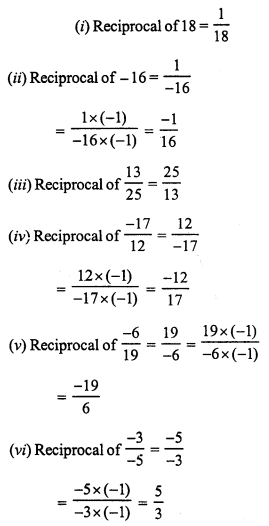(vii) Reciprocal of -1 = -1
(viii) Reciprocal of 0 does not exist.

Question 2.
Solution: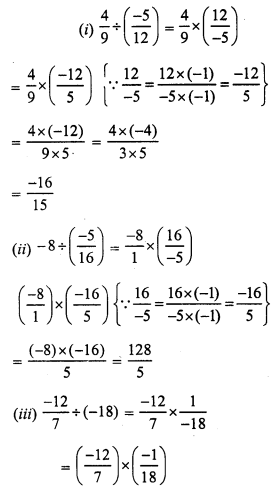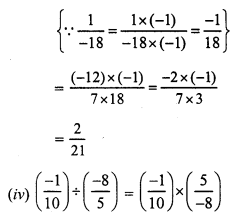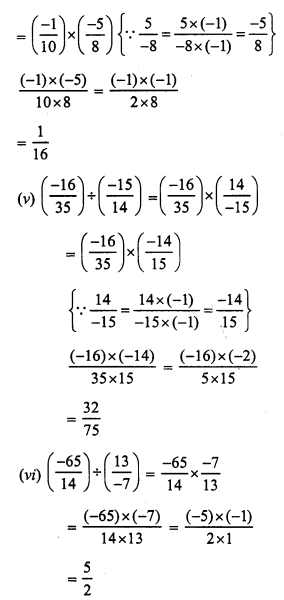Question 3.
Solution: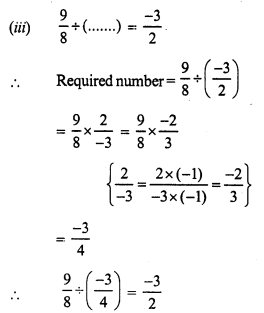Question 4.
Solution:Question 5.
Solution: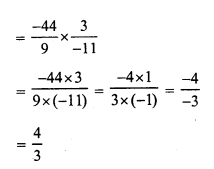Question 6.
Solution: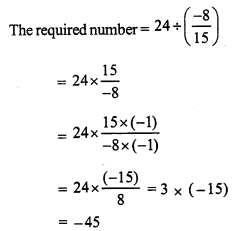Question 7.
Solution:
Product of two number = 10
One number = -8
Second number = 10 ÷ (-8)Question 8.
Solution:
Product of two rational numbers = – 9
One number = -12
Second number = (-9) ÷ (-12)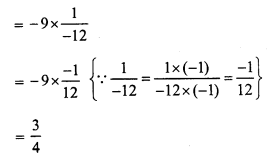Question 9.
Solution:Question 10.
Solution: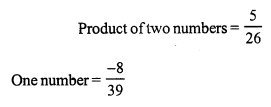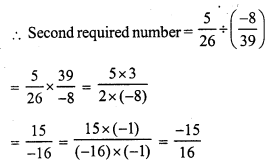Question 11.
Solution:
Cloth required for 24 pairs of trousers =54 m
Cloth required for one pair = (54 ÷ 24) m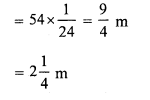Question 12.
Solution:
Total length of rape = 30 m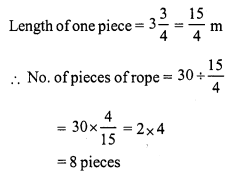Question 13.
Solution: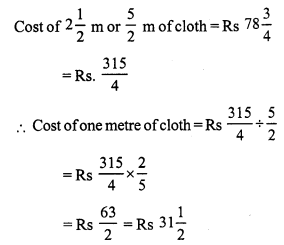Hope given RS Aggarwal Solutions Class 7 Chapter 4 Rational Numbers Ex 4F are helpful to complete your math homework.

If you have any doubts, please comment below. Learn Insta try to provide online math tutoring for you.